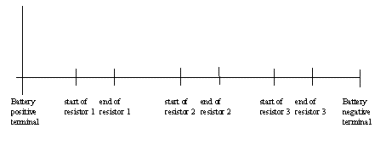Teaching Physics with the Physics Suite Edward F. Redish Problems Sorted by Type | Problems Sorted by Subject | Problems Sorted by Chapter in UP

Tracking round a circuit

 The circuit shown in the diagram at the right contains a battery and 3 resistors. The battery has an EMF of 5 V, R1 = 2 Ω, R2 = 3 Ω, and R3 =Ā5 Ω. Below are shown 3 graphs tracking some quantity around the circuit. On the first, plot the voltage a test charge would experience as it moved throughout the circuit. On the second, plot the electric field a test charge would experience as it moved through the circuit. On the third, plot the current one would measure crossing a plane perpendicular to the wire of the circuit as one goes through the circuit.Not finding what you wanted? Check the Site Map for more information.